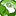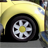# QlikView App Dev

Discussion Board for collaboration related to QlikView App Development.

Announcements
cancel
Showing results for
Did you mean:Contributor II

## how do I create custom fields after binary load?

Hello again, and thanks for helping out.

I'm doing a binary load, and then after this, I need to make some custom fields. These custom fields worked when I was not using the binary load, but instead I was loading an Excel file. Now I have to use the binary load to link to my company's other dashboards.  Here's what I currently have:

Binary: \\pathnamehere\datamodel.qvw;

Then on another tab, I have:

concatenate (Projects)

// // Button fields //
if( month(Created_Date)= month(Today()) and Year(Created_Date)=year(Today()),1) as CreatedThisMonth,
if( month([Actual start date])= month(Today()) and Year([Actual start date])=year(Today()),1) as ActualStartThisMonth,
if( month([Actual end date])= month(Today()) and Year([Actual end date])=year(Today()),1) as ActualEndThisMonth,
if( month([Planned start date])= month(Today()) and Date(Year([Planned start date]))=year(Today()),1) as PlannedStartThisMonth,
if( month([Planned end date])= month(Today()) and Year([Planned end date])=year(Today()),1) as PlannedEndThisMonth,
if( month([Actual end date]) <= month(Today()) and Year([Actual end date])<=year(Today()),1) as EndEarly,
if( month([Actual start date]) <= month(Today()) and Year([Actual start date])<=year(Today()),1) as StartEarly,
if([Overall health] = 'Red' and (State = 'Work in Progress' or State = 'On Hold') , 1) as ActiveRed,
if([Overall health] = 'Yellow' and (State = 'Work in Progress' or State = 'On Hold') , 1) as ActiveYellow,
if([Overall health] = 'Green' and (State = 'Work in Progress' or State = 'On Hold') , 1) as ActiveGreen,
if([Missing_Status] = 'Yes' and (State = 'Work in Progress' or State = 'On Hold') , 1) as ActiveMissingStatus
// // End Button fields //

Resident Projects;

So ... what am I doing wrong?

Also, the fields [Created_Date and Actual start date]  were date fields in my Excel, but are number fields in the binary load.

Thanks

AJ

2 RepliesSpecialist

Is Projects table available in the datamodel.qvw??

You Can try this

ProjectMain:

*,

if( month(Created_Date)= month(Today()) and Year(Created_Date)=year(Today()),1) as CreatedThisMonth,

if( month([Actual start date])= month(Today()) and Year([Actual start date])=year(Today()),1) as ActualStartThisMonth,

if( month([Actual end date])= month(Today()) and Year([Actual end date])=year(Today()),1) as ActualEndThisMonth,

if( month([Planned start date])= month(Today()) and Date(Year([Planned start date]))=year(Today()),1) as PlannedStartThisMonth,

if( month([Planned end date])= month(Today()) and Year([Planned end date])=year(Today()),1) as PlannedEndThisMonth,

if( month([Actual end date]) <= month(Today()) and Year([Actual end date])<=year(Today()),1) as EndEarly,

if( month([Actual start date]) <= month(Today()) and Year([Actual start date])<=year(Today()),1) as StartEarly,

if([Overall health] = 'Red' and (State = 'Work in Progress' or State = 'On Hold') , 1) as ActiveRed,

if([Overall health] = 'Yellow' and (State = 'Work in Progress' or State = 'On Hold') , 1) as ActiveYellow,

if([Overall health] = 'Green' and (State = 'Work in Progress' or State = 'On Hold') , 1) as ActiveGreen,

if([Missing_Status] = 'Yes' and (State = 'Work in Progress' or State = 'On Hold') , 1) as ActiveMissingStatus

Resident Projects;

Drop table  Projects;Contributor II
Author

Hi Rupam

Yes, the Projects table exists. This worked - basically I made a new table to hold the button fields, rather than trying to add it to the existing Projects table. Only thing I did differently is to not drop the Projects table, as it had more info I still needed. By including the project number I was able to create a link between them.

thanks !!

AJ